# MP Board Class 7th Maths Solutions Chapter 4 Simple Equations Ex 4.4

## MP Board Class 7th Maths Solutions Chapter 4 Simple Equations Ex 4.4

Question 1.
Set up equations and solve them to find the unknown numbers in the following cases:
(a) Add 4 to eight times a number; you get 60.
(b) One-fifth of a number minus 4 gives 3.
(c) If I take three-fourths of a number and add 3 to it, I get 21.
(d) When I subtracted 11 from twice a number, the result was 15.
(e) Munna subtracts thrice the number of notebooks he has from 50, he finds the result to be 8.
(f) Ibenhal thinks of a number. If she adds 19 to it and divides the sum by 5, she will get 8.
(g) Anwar thinks of a number. If he takes away 7 from $$\frac{5}{2}$$ of the number, the result is 23.
Solution:
(a) Let the number be x.
8 times of this number = 8x
According to question;
8x + 4 = 60 ⇒ 8x = 60 – 4
(Transposing 4 to R.H.S.)
⇒ 8x = 56
Dividing both sides by 8,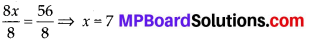(b) Let the number be x
One-fifth of this number $$\frac{x}{5}$$
According to question;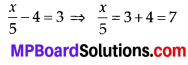(Transporting -4 to R.H.S.)
Mutiplying both sides by 5,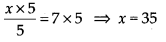(c) Let the number be x.
Three-fourth of this number = $$\frac{3 x}{4}$$
According to question;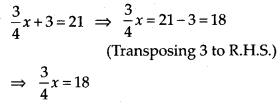Multiplying both sides by 4,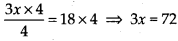Dividing both sides by 3,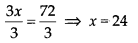(d) Let the number be x.
Twice of this number = 2x
According to question;
2x – 11 = 15 ⇒ 2x = 15 + 11
(Transposing -11 to R.H.S.)
⇒ 2x = 26
Dividing both sides by 2,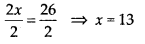(e) Let the number of notebooks be x.
Thrice the number of notebooks = 3x
According to question;
⇒ 50 – 3x = 8 ⇒ – 3x = 8 – 50
(Transposing 50 to R.H.S.)
⇒ – 3x = – 42
Dividing both sides by – 3,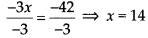(f) Let the number be x.
According to question;Multiplying both sides by 5,⇒ x = 40 – 19 (Transposing 19 to R.H.S.)
⇒ x = 21

(g) Let the number be x.
$$\frac{5}{2}$$ of this number = $$\frac{5x}{2}$$
According to question;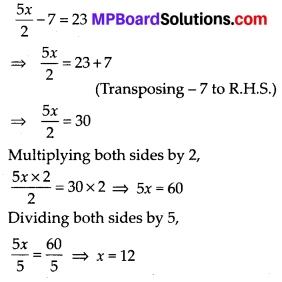Question 2.
Solve the following;
(a) The teacher tells the class that the highest marks obtained by a student in her class is twice the lowest marks plus 7. The highest score is 87. What is the lowest score?
(b) In an isosceles triangle, the base angles are equal. The vertex angle is 40°. What are the base angles of the triangle? (Remember, the sum of three angles of a triangle is 180°).
(c) Sachin scored twice as many runs as Rahul. Together, their runs fell two short of a double century. How many runs did each one score?
Solution:
(a) Let the lowest score be l.
According to question;
Highest marks = 2 × Lowest marks + 7
⇒ 87 = (2 × l) + 7 × 2l + 7 = 87
⇒ 2l = 87 – 7
(Transposing 7 to R.H.S.)
⇒ 2l = 80
Dividing both sides by 2,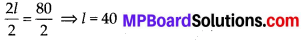Therefore, the lowest score is 40.

(b) Let the base angles be equal to b.
Vertex angle = 40°
The sum of all interior angles of a triangle is 180°.
b + b + 40° = 180° ⇒ 2b + 40° = 180°
⇒ 2b = 180° – 40° = 140°
(Transposing 40° to R.H.S.)
Dividing both sides by 2,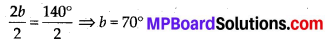Therefore, the base angles of the triangle are of 70°.

(c) Let Rahul’s score be x.
Therefore, Sachin’s score = 2x
According to question;
Rahul’s score + Sachin’s score = 200 – 2
2x + x = 198 ⇒ 3x = 198
Dividing both sides by 3,∴ Rahul’s score = 66
Sachin’s score = 2 × 66 = 132Question 3.
Solve the following:
(i) Irfan says that he has 7 marbles more than five times the marbles Parmit has. Irfan has 37 marbles. How many marbles does Parmit have?
(ii) Laxmi’s father is 49 years old. He is 4 years older than three times Laxmi’s age. What is Laxmi’s age?
(iii) People of Sundargram planted trees in the village garden. Some of the trees were fruit trees. The number of non-fruit trees were two more than three times the number of fruit trees. What was the number of fruit trees planted if the number of non-fruit trees planted was 77?
Solution:
(i) Let Parmit has x marbles.
5 times the number of marbles Parmit has = 5x
According to question;
5x + 7 = 37 ⇒ 5x = 37 – 7 = 30
(Transposing 7 to R.H.S.)
Dividing both sides by 5,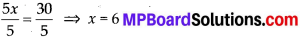Therefore, Parmit has 6 marbles.

(ii) Let Laxmi’s age be x years.
According to question;
Her father’s age = 3 × Laxmi’s age + 4
⇒ 49 = (3 × x) + 4
⇒ 3x + 4 = 49 ⇒ 3x = 49 – 4
(Transposing 4 to R.H.S.)
⇒ 3x = 45
Dividing both sides by 3,Therefore, Laxmi’s age is 15 years.

(iii) Let the number of fruit trees be x. According to question;
Number of non-fruit trees = 3 × Number of fruit trees + 2
77 = (3 × x) + 2
⇒ 3x + 2 = 77 ⇒ 3x = 77 – 2
(Transposing 2 to R.H.S.)
⇒ 3x = 75
Dividing both sides by 3,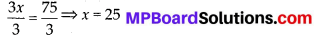Therefore, the number of fruit trees planted were 25.

Question 4.
Solve the following riddle: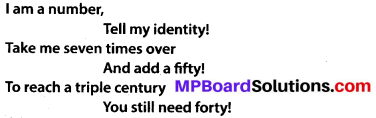Solution:
Let the number be x.
Seven times x = 7x
According to question; .
(7x + 50) + 40 = 300 ⇒ 7x + 90 = 300
⇒ 7x = 300 – 90 (Transposing 90 to R.H.S.)
⇒ 7x = 210
Dividing both sides by 7,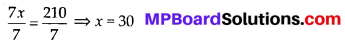Therefore, the number is 30.